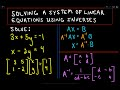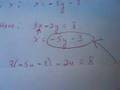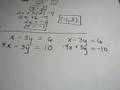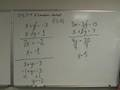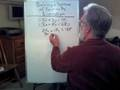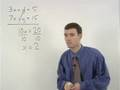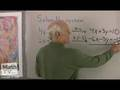Algebraic Methods of Solving a Pair of Linear Equations  ( 0)

Questions

Q. A two digit number is obtained by either multiplying the sum of the digits by 8 and adding 1, or by multiplying the difference of ... 1, 001 answer under Algebraic Methods of... 8 years, 2 months ago

A. Let xy be the unknown number. ==&gt; x = tens digit, and y = ones digit. Then from the given, 8(x+y) + 1= 10x + y, and 13(x-y) + 2 = 10x + y. &lt;==&gt; 2x - 7y = 1 and -3x + 14y = 2 ... link
8 years, 2 months ago